# Equivalence Points

aqion
Aug. 15, 2017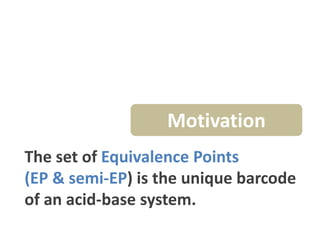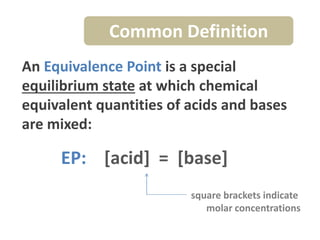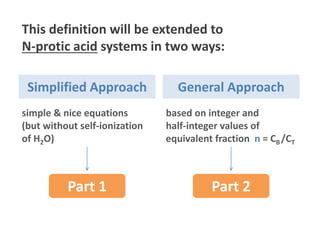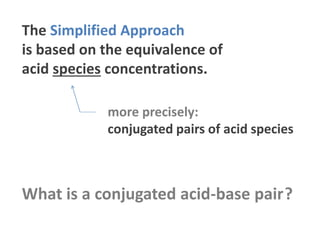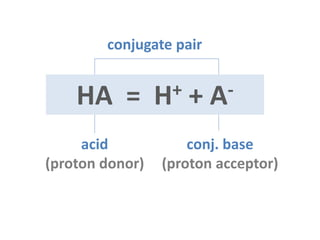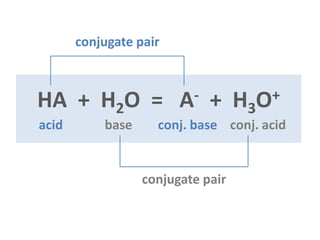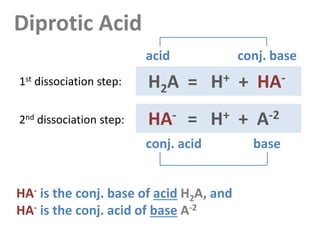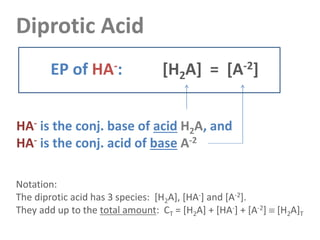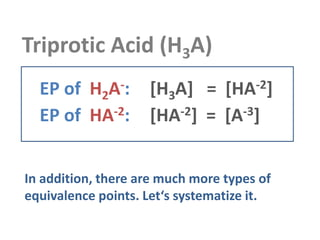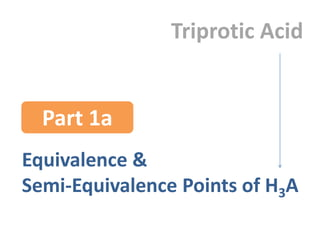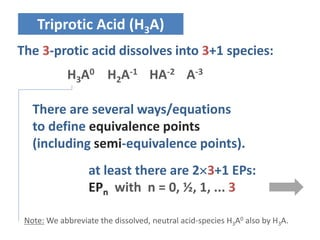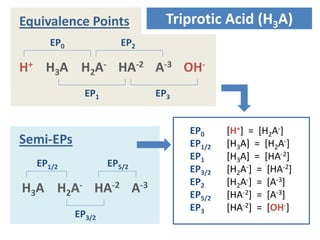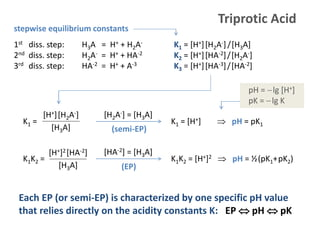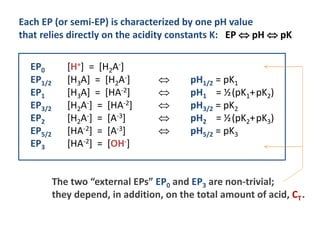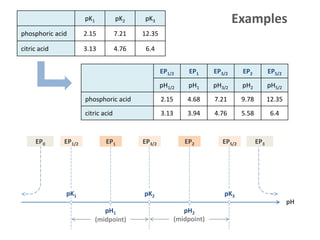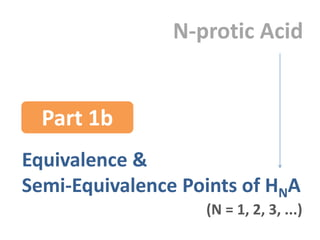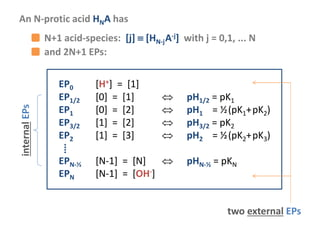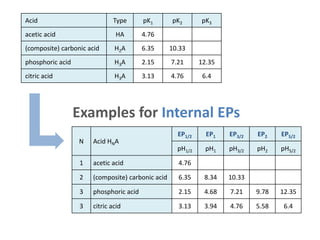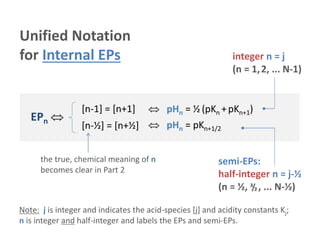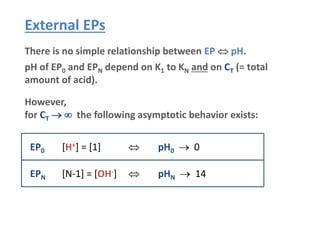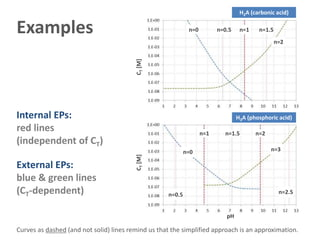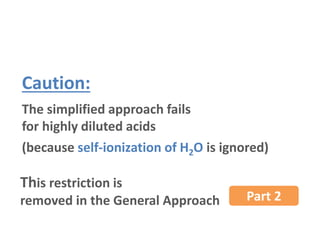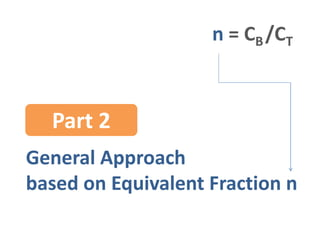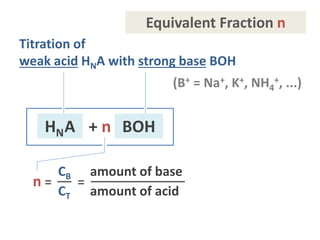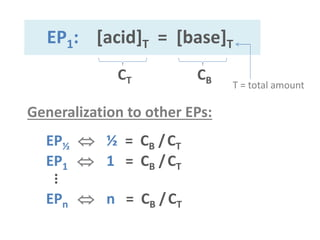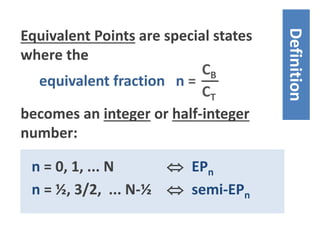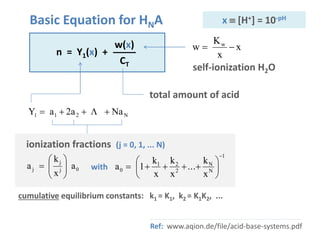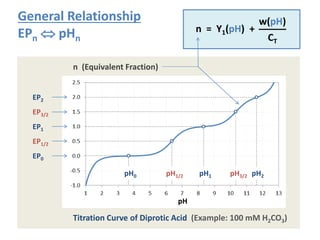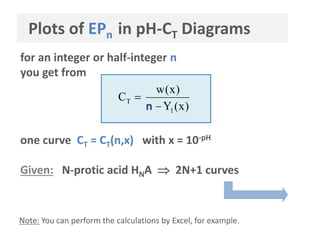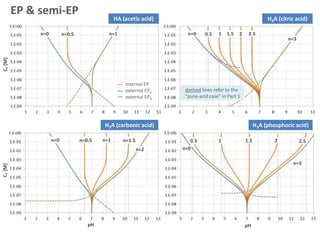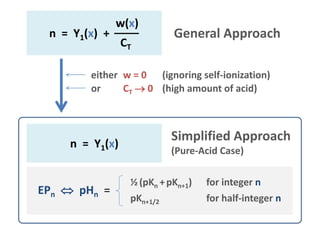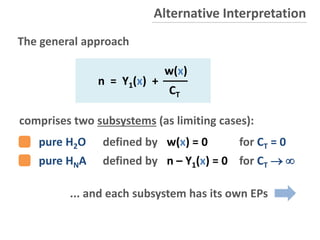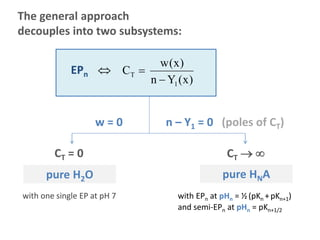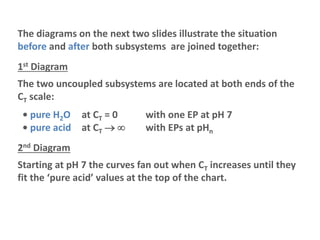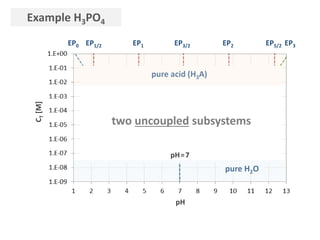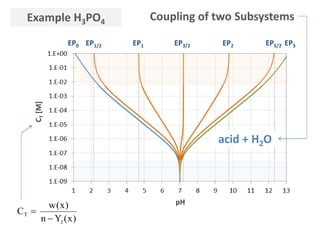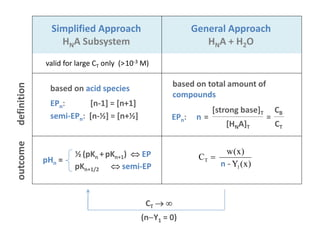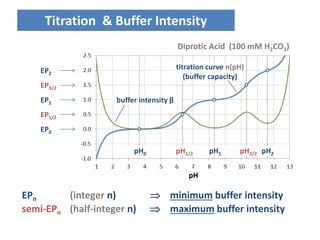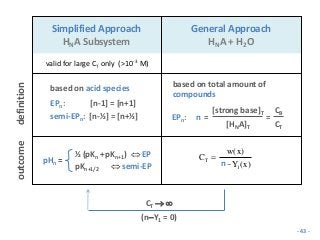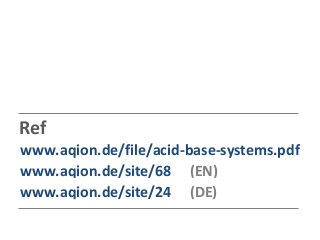1 of 44

### Equivalence Points

• 1. Equivalence Points (EP) Systematics & Classification aqion.de updated 2017-09-28
• 2. The set of Equivalence Points (EP & semi-EP) is the unique barcode of an acid-base system. Motivation - 2 -
• 3. An Equivalence Point is a special equilibrium state at which chemical equivalent quantities of acids and bases are mixed: EP: [acid] = [base] square brackets indicate molar concentrations Common Definition - 3 -
• 4. This definition will be extended to N-protic acid systems in two ways: Part 1 Simplified Approach General Approach Part 2 simple & nice equations (but without self-ionization of H2O) based on integer and half-integer values of equivalent fraction n = CB /CT - 4 -
• 5. Simplified Approach (valid for High-Concentrated Acids) Part 1
• 6. The Simplified Approach is based on the equivalence of acid species concentrations. more precisely: conjugated pairs of acid species What is a conjugated acid-base pair? - 6 -
• 7. acid (proton donor) conj. base (proton acceptor) conjugate pair HA = H+ + A- - 7 -
• 8. HA + H2O = A- + H3O+ conjugate pair conjugate pair acid base conj. base conj. acid - 8 -
• 9. Diprotic Acid H2A = H+ + HA- HA- = H+ + A-2 conj. acid base acid conj. base 1st dissociation step: 2nd dissociation step: HA- is the conj. base of acid H2A, and HA- is the conj. acid of base A-2 - 9 -
• 10. HA- is the conj. base of acid H2A, and HA- is the conj. acid of base A-2 EP of HA-: [H2A] = [A-2] Diprotic Acid Notation: The diprotic acid has 3 species: [H2A], [HA-] and [A-2]. They add up to the total amount: CT = [H2A] + [HA-] + [A-2]  [H2A]T
• 11. Triprotic Acid (H3A) EP of H2A-: [H3A] = [HA-2] EP of HA-2: [HA-2] = [A-3] In addition, there are much more types of equivalence points. Let‘s systematize it. - 11 -
• 12. Equivalence & Semi-Equivalence Points of H3A Part 1a Triprotic Acid
• 13. Triprotic Acid (H3A) The 3-protic acid dissolves into 3+1 species: H3A0 H2A-1 HA-2 A-3 There are several ways/equations to define equivalence points (including semi-equivalence points). at least there are 23+1 EPs: EPn with n = 0, ½, 1, ... 3 Note: We abbreviate the dissolved, neutral acid-species H3A0 also by H3A.
• 14. Triprotic Acid (H3A) EP0 [H+] = [H2A-] EP1/2 [H3A] = [H2A-] EP1 [H3A] = [HA-2] EP3/2 [H2A-] = [HA-2] EP2 [H2A-] = [A-3] EP5/2 [HA-2] = [A-3] EP3 [HA-2] = [OH-] H+ H3A H2A- HA-2 A-3 OH- EP0 EP2 EP1 EP3 Equivalence Points H3A H2A- HA-2 A-3 EP1/2 EP5/2 EP3/2 Semi-EPs
• 15. Triprotic Acid 1st diss. step: H3A = H+ + H2A- K1 = [H+][H2A-]/[H3A] 2nd diss. step: H2A- = H+ + HA-2 K2 = [H+][HA-2]/[H2A-] 3rd diss. step: HA-2 = H+ + A-3 K3 = [H+][HA-3]/[HA-2] stepwise equilibrium constants K1 = K1 = [H+]  pH = pK1 [H2A-] = [H3A] (semi-EP) [H+][H2A-] [H3A] K1K2 = K1K2 = [H+]2  pH = ½(pK1+pK2) [HA-2] = [H3A] (EP) [H+]2[HA-2] [H3A] Each EP (or semi-EP) is characterized by one specific pH value that relies directly on the acidity constants K: EP  pH  pK pH = lg [H+] pK = lg K
• 16. EP0 [H+] = [H2A-] EP1/2 [H3A] = [H2A-]  pH1/2 = pK1 EP1 [H3A] = [HA-2]  pH1 = ½(pK1+pK2) EP3/2 [H2A-] = [HA-2]  pH3/2 = pK2 EP2 [H2A-] = [A-3]  pH2 = ½(pK2+pK3) EP5/2 [HA-2] = [A-3]  pH5/2 = pK3 EP3 [HA-2] = [OH-] Each EP (or semi-EP) is characterized by one pH value that relies directly on the acidity constants K: EP  pH  pK The two “external EPs” EP0 and EP3 are non-trivial; they depend, in addition, on the total amount of acid, CT. - 16 -
• 17. EP1/2 EP1 EP3/2 EP2 EP5/2 pH1/2 pH1 pH3/2 pH2 pH5/2 phosphoric acid 2.15 4.68 7.21 9.78 12.35 citric acid 3.13 3.94 4.76 5.58 6.4 pK1 pK2 pK3 phosphoric acid 2.15 7.21 12.35 citric acid 3.13 4.76 6.4 pK1 pK2 EP1/2 EP1 pK3 pH EP5/2EP2EP3/2 (midpoint) (midpoint) pH1 pH2 EP0 EP3 Examples - 17 -
• 18. Equivalence & Semi-Equivalence Points of HNA Part 1b N-protic Acid (N = 1, 2, 3, ...)
• 19. EP0 [H+] =  EP1/2  =   pH1/2 = pK1 EP1  =   pH1 = ½(pK1+pK2) EP3/2  =   pH3/2 = pK2 EP2  =   pH2 = ½(pK2+pK3) ⁞ EPN-½ [N-1] = [N]  pHN-½ = pKN EPN [N-1] = [OH-] An N-protic acid HNA has internalEPs two external EPs and 2N+1 EPs: N+1 acid-species: [j]  [HN-jA-j] with j = 0,1, ... N
• 20. N Acid HNA EP1/2 EP1 EP3/2 EP2 EP5/2 pH1/2 pH1 pH3/2 pH2 pH5/2 1 acetic acid 4.76 2 (composite) carbonic acid 6.35 8.34 10.33 3 phosphoric acid 2.15 4.68 7.21 9.78 12.35 3 citric acid 3.13 3.94 4.76 5.58 6.4 Acid Type pK1 pK2 pK3 acetic acid HA 4.76 (composite) carbonic acid H2A 6.35 10.33 phosphoric acid H3A 2.15 7.21 12.35 citric acid H3A 3.13 4.76 6.4 Examples for Internal EPs
• 21. Unified Notation for Internal EPs EPn   pHn = ½ (pKn +pKn+1)  pHn = pKn+1/2 [n-1] = [n+1] [n-½] = [n+½] integer n = j (n = 1,2, ... N-1) half-integer n = j-½ (n = ½, ³/₂, ... N-½) semi-EPs: Note: j is integer and indicates the acid-species [j] and acidity constants Kj; n is integer and half-integer and labels the EPs and semi-EPs. the true, chemical meaning of n becomes clear in Part 2
• 22. External EPs EPN [N-1] = [OH-]  pHN  14 EP0 [H+] =   pH0  0 There is no simple relationship between EP  pH. pH of EP0 and EPN depend on K1 to KN and on CT (= total amount of acid). However, for CT   the following asymptotic behavior exists: - 22 -
• 23. pH CT[M]CT[M] n=0 n=0.5 n=1.5n=1 n=2 n=0 n=0.5 n=1.5n=1 n=2 n=2.5 n=3 H3A (phosphoric acid) H2A (carbonic acid) Examples Internal EPs: red lines (independent of CT) External EPs: blue & green lines (CT-dependent) Curves as dashed (and not solid) lines remind us that the simplified approach is an approximation.
• 24. Caution: The simplified approach fails for highly diluted acids This restriction is removed in the General Approach Part 2 (because self-ionization of H2O is ignored)
• 25. General Approach based on Equivalent Fraction n Part 2 n = CB/CT
• 26. (B+ = Na+, K+, NH4 +, ...) n = = CB amount of base CT amount of acid HNA BOH+ n Titration of weak acid HNA with strong base BOH Equivalent Fraction n - 26 -
• 27. EP1  1 = CB /CT EP½  ½ = CB /CT EP1: [acid]T = [base]T Generalization to other EPs: EPn  n = CB /CT ⁞ CT CB T = total amount - 27 -
• 28. equivalent fraction n = CB CT Equivalent Points are special states where the n = 0, 1, ... N  EPn n = ½, 3/2, ... N-½  semi-EPn Definition becomes an integer or half-integer value: - 28 -
• 29. Basic Equation for HNA n = Y1(x) + w(x) CT self-ionization H2O x x K w w  N211 Naa2aY   0j j j a x k a        1 N N 2 21 0 x k ... x k x k 1a         ionization fractions (j = 0, 1, ... N) with cumulative equilibrium constants: k1 = K1, k2 = K1K2, ... total amount of acid x  [H+] = 10-pH Ref: www.aqion.de/file/acid-base-systems.pdf
• 30. Plots of EPn in pH-CT Diagrams for an integer or half-integer n you get from one curve CT = CT(n,x) with x = 10-pH )x(Yn )x(w C 1 T   n Given: N-protic acid HNA  2N+1 curves Note: You can perform the calculations by Excel, for example. - 30 -
• 31. General Relationship EPn  pHn pH n (Equivalent Fraction) pH1/2 pH3/2pH0 pH1 pH2 EP1/2 EP3/2 EP0 EP1 EP2 Titration Curve of Diprotic Acid (Example: 100 mM H2CO3) n = Y1(pH) + w(pH) CT
• 32. n=0 n=0.5 n=1 n=0 n=0.5 n=1 n=1.5 n=2 HA (acetic acid) H2A (carbonic acid) CT[M] n=0 0.5 1.51 2 2.5 n=3 H3A (phosphoric acid) n=0 0.5 1.51 2 2.5 n=3 H3A (citric acid) pH CT[M] pH EP & semi-EP internal EP external EP0 external EPN dashed lines refer to the “pure-acid case” in Part 1
• 33. n = Y1(x) + w(x) CT General Approach n = Y1(x) Simplified Approach (Pure-Acid Case) either w = 0 (ignoring self-ionization) or CT  0 (high amount of acid) EPn  pHn = ½ (pKn +pKn+1) for integer n pKn+1/2 for half-integer n
• 34. comprises two subsystems (as limiting cases): pure H2O defined by w(x) = 0 for CT = 0 pure HNA defined by n – Y1(x) = 0 for CT   n = Y1(x) + w(x) CT The general approach Alternative Interpretation ... and each subsystem has its own EPs - 34 -
• 35. The general approach decouples into two subsystems: CT = 0 CT   pure H2O pure HNA with one single EP at pH 7 with EPn at pHn = ½(pKn +pKn+1) and semi-EPn at pHn = pKn+1/2 w = 0 n – Y1 = 0 (poles of CT) )x(Yn )x(w C 1 T  EPn  - 35 -
• 36. The diagrams on the next two slides illustrate the situation before and after both subsystems are joined together: 1st Diagram The two uncoupled subsystems are located at both ends of the CT scale: • pure H2O at CT = 0 with one EP at pH 7 • pure acid at CT   with EPs at pHn 2nd Diagram Starting at pH 7 the curves fan out when CT increases until they fit the ‘pure acid’ values at the top of the chart. - 36 -
• 37. Example H3PO4 CT[M] pure acid (H3A) pure H2O two uncoupled subsystems pH pH=7 EP1/2 EP3/2EP1 EP2 EP5/2EP0 EP3 - 37 -
• 38. Example H3PO4 CT[M] acid + H2O pH EP1/2 EP3/2EP1 EP2 EP5/2EP0 EP3 )x(Yn )x(w C 1 T   Coupling of two Subsystems - 38 -
• 39. Summary
• 40. An N-protic acid has 2N+1 equivalence points: EPn  n = CB /CT for n = 0, ½, 1, ... N Equivalence points are special equilibrium states where the equivalent fraction n = CB/CT becomes an integer or half-integer value. The relationship EPn  pHn is given by where Y1 describes the acid and w the water. n = Y1(pH) + w(pH)/CT For high-concentrated acids (CT  ) the relationship simplifies to: n = Y1(pH) which yields the direct link to acidity constants: ½ (pKn +pKn+1)  EP pKn+1/2  semi-EP pHn =
• 41. For high-concentrated acids (CT  ) there is an alternative definition of EPs based on equal species concentrations: EPn: [n-1] = [n+1] semi-EPn: [n-½] = [n+½] Example: In carbonate systems EP1 is often introduced as state where [H2CO3] = [CO3 -2]. The equivalent fraction n = Y1+ w/CT (titration curve) describes the buffer capacity. Its pH-derivative is the buffer intensity β = dn/dpH. EPs are extrema of β: EPn  minimum buffer intensity semi-EPn  maximum buffer intensity
• 42. Titration & Buffer Intensity EPn (integer n)  minimum buffer intensity semi-EPn (half-integer n)  maximum buffer intensity pH titration curve n(pH) (buffer capacity) buffer intensity β EP1/2 EP3/2 EP0 EP1 EP2 pH1/2 pH3/2pH0 pH1 pH2 Diprotic Acid (100 mM H2CO3)
• 43. Simplified Approach HNA Subsystem General Approach HNA + H2O valid for large CT only (>10-3 M) definition based on acid species EPn: [n-1] = [n+1] semi-EPn: [n-½] = [n+½] outcome ½ (pKn +pKn+1)  EP pKn+1/2  semi-EP pHn = CT   (nY1 = 0) )x(Yn )x(w C 1 T   n based on total amount of compounds EPn: n = = [HNA]T [strong base]T CT CB - 43 -
• 44. Ref www.aqion.de/file/acid-base-systems.pdf www.aqion.de/site/68 (EN) www.aqion.de/site/24 (DE)